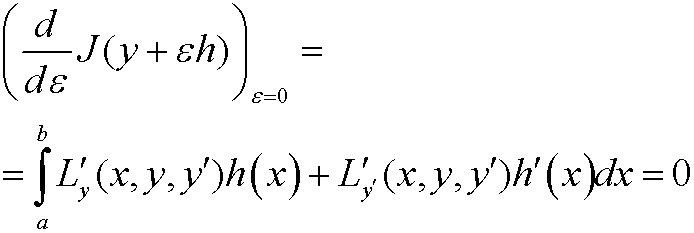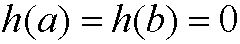8. Proof of theorem 1.

We need the fundamental lemma of calculus of variations:

Lemma: Let f be a function in C[a,b] and assume thatfor everyThenProof of theorem 1: Let y be an extremal and letThenis an admissible and competing function forConsider the functionalSince y  is an extremal we must haveIf we move the derivative inside the integral we getThis means thatfor allWe integrate the second term by parts and get<>Since

<>this implies thatWe thus havefor allIf we finally use the lemma above we can conclude thatThe proof is complete.